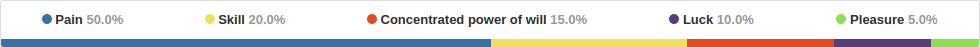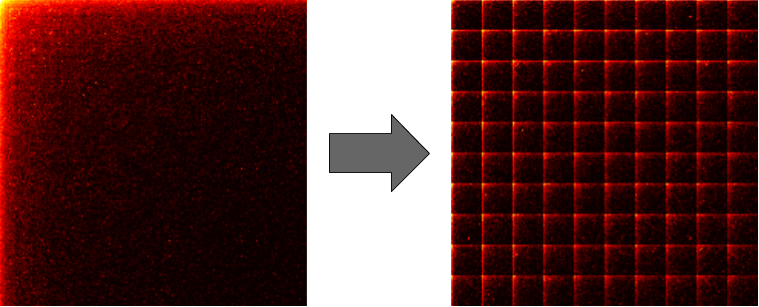# Similarity of GitHub Repositories by Source Code Identifiers

## Objective

Given a single software repository, find repositories which are related.

"Related" is subjective.

"Related" = "similar" here.

## Ways to find similar repos

1. Collaborative filtering
2. Content analysis

Egor will talk about (1) after lunch.

We will address (2) in this presentation.

## Plan

1. Turn each repository into features.
2. Apply some algorithm to search for the NN.
0 0 1 0 1 0
?
1 0 1 0 1 0
0 0 0 1 0 0
0 1 0 0 1 0

## What is source code?

```		`def nearest_neighbors(self, origin, k=10, early_stop=0.5, max_time=3600,`
`                      skipped_stop=0.99, throw=True):`
`    # origin can be either a text query or an id`
`    if isinstance(origin, (tuple, list)):`
`	     words, weights = origin`
`	     weights = numpy.array(weights, dtype=numpy.float32)`
`	     index = None`
`	     avg = self._get_centroid(words, weights, force=True)`
`    else:`
`	     index = origin`
`	     words, weights = self._get_vocabulary(index)`
`	     avg = self._get_centroid_by_index(index)`
`    if avg is None:`
`	     raise ValueError(`
`		     "Too little vocabulary for %s: %d" % (index, len(words)))`
```
```				`⬤ keywords`
`⬤ identifiers`
`⬤ literals`
`⬤ strings`
`⬤ comments`
```

## Points of fun

• Development history
• Project structure
• Language vector
• Source code structure
• Identifiers

## Development history

Mining artifacts from VCS (git).

Finding repositories which have a similar evolution or development culture.

E.g. comparing projects by maturity.

source{d}'s dataset.

## Project structure

Comparing file system trees.

Finding repositories which have a similar structure.

E.g. Wordpress web sites.

## Language vector

Aka GitHub language bars.Finding projects of the same alignment.

E.g. C autotools != C cmake.

source{d}'s dataset.

Pure NLP and entity extraction.

Finding projects with similar descriptions / topics.

E.g. all projects related to Bitcoin.

source{d}'s dataset.

## Source code structure

Based on ASTs: source{d}'sproject Babelfish.

Finding projects with similar algorithms.

E.g. projects which implement quick sort.

"short-distance" NLP.

Sentiment analysis. Finding projects where developers are happy or sad.

Finding projects where developers have similar vocabulary.

Native human language detection.

## Identifiers

One of the richest features.

They carry NLP and algorithmic payloads.

This talk focuses on them.

source{d}'s dataset.

## Parsing at scale

In pre-Babelfish era, we have to separately classify and regexp parse sources.

Classification: github/linguist, src-d/simple-linguist.

Parsing: Pygments, highlight.js.

Perfectly matches to Spark.

## Identifier extraction

We use Pygments:

`Token.Name.*`

Try yourself:

`pip3 install pygments`
`pygmentize -f raw /path/to/file`

## Identifier extraction

We split identifiers:

• `UpperCamelCase -> (upper, camel, case)`
• `camelCase -> (camel, case)`
• `FRAPScase -> (fraps, case)`
• `SQLThing -> (sql, thing)`
• `_Astra -> (astra)`
• `CAPS_CONST -> (caps, const)`
• `_something_SILLY_ -> (something, silly)`
• `blink182 -> (blink)`
• `FooBar100500Bingo -> (foo, bar, bingo)`
• `Man45var -> (man, var)`
• `method_name -> (method, name)`
• `Method_Name -> (method, name)`
• `101dalms -> (dalms)`
• `101_dalms -> (dalms)`
• `101_DalmsBug -> (dalms, bug)`
• `101_Dalms45Bug7 -> (dalms, bug)`
• `wdSize -> (size, wdsize)`
• `Glint -> (glint)`
• `foo_BAR -> (foo, bar)`

Algorithm on GitHub.

## Identifier extraction

Finally, we apply Snowball stemmer to words longer than 6 chars.

## Weighted nBOW

Let's interpret every repository as the weighted bag-of-words.

We calculate TF-IDF to weight the occurring identifiers.

`tensorflow/tensorflow:`
```    tfreturn	67.780249
oprequires	63.968142
doblas	63.714424
gputools	62.337396
tfassign	61.545926
opkernel	60.721556
sycl	57.064558
hlo	55.723587
libxsmm	54.820668
tfdisallow	53.666890```

## Topic modeling

We can run topic modeling on these features.

Post on Habrahabr.

Paper on ArXiV - accepted to AI'17 (Sydney).

Topic #36 "Movies" →

## Identifier embeddings

tensorflow float unaligned vector ttypes types const unaligned vec int unaligned vector ttypes types int const unaligned vec example statistics norm normalized squared regularizations regularizations status initialize construction kernel opkernel context error iferror return tfreturn context attr get symmetric error iferror return tfreturn context attr get symmetric shrinkage symmetric symmetric status shrink weight shrinked std max std abs weight shrinkage shrinked std copysign shrinked weight eigen tensor eigen major row eigen shrink eigen tensor eigen major row weights weights sign weights abs weights constant shrinkage cwise max weights constant shrinkage cwise max weights constant symmetric symmetric symmetric symmetric shrinkage and assign copy disallow tfdisallow regularizations model weights example example statistics and compute example norm weighted wxand num partitions model weights model weights regularizations regularization example label example label example weight example weight norm squared norm squared features sparse std ptr unique int unaligned vector indices std ptr unique float unaligned vector values std vector features sparse features sparse dense vector eigen map tensor eigen tensor eigen major row row eigen map tensor eigen tensor eigen major row data matrix data index row data matrix dimension data matrix dimension ttypes types const matrix data matrix int index row std vector std ptr unique dense vector dense vectors example label example weight norm squared examples model weights model weights model weights delta update weights eigen device pool thread device example example bounded delta dual normalized sparse weights size example features sparse features sparse example features sparse feature weights feature weights sparse weights int features sparse indices size feature value features sparse values features sparse values feature weights deltas features sparse indices feature value bounded delta dual normalized dense weights size example dense vector dense vector example dense vectors ttypes types vec deltas dense weights deltas deltas device device deltas dense vector row deltas constant bounded delta dual normalized status initialize context kernel opkernel context input list opinput inputs sparse weights error iferror return tfreturn context input list inputs sparse weights input list opinput dense inputs weights error iferror return tfreturn context input list dense inputs weights list opoutput output outputs sparse weights error iferror return tfreturn context list output outputs sparse weights list opoutput output dense outputs weights error iferror return tfreturn context list output dense outputs weights intialize weights input list opinput inputs weight list opoutput output outputs weight std vector feature weights feature weights inputs weight size tensor delta outputs weight allocate inputs weight shape delta deltas delta flat deltas set zero feature weights back emplace feature weights inputs weight flat deltas intialize weights inputs sparse weights outputs sparse weights sparse weights intialize weights dense inputs weights dense outputs weights dense weights status feature weights ttypes types vec nominals ttypes types vec deltas std vector feature weights sparse weights std vector feature weights dense weights example and assign copy disallow tfdisallow model weights example statistics example and compute example norm weighted wxand num partitions model weights model weights regularizations regularization example statistics result result norm normalized squared norm squared regularization symmetric features sparse size example features sparse features sparse features sparse model weights feature weights sparse weights model weights sparse weights int features sparse indices size int feature index features sparse indices feature value features sparse values features sparse values feature weight sparse weights nominals feature index sparse weights deltas feature index num partitions result feature value regularization shrink feature weight dense vectors size example dense vector dense vector dense vectors model weights feature weights dense weights model weights dense weights eigen tensor eigen major row feature weights dense weights nominals dense weights deltas dense weights deltas constant num partitions eigen tensor eigen major row prediction dense vector row regularization eigen shrink feature weights sum result prediction result examples examples example example example index examples example index examples num examples size features num features num status initialize context kernel opkernel context features num sparse features num sparse values with dense features num features num features num sparse dense features num input list opinput example indices inputs sparse error iferror return tfreturn context input list example indices inputs sparse input list opinput feature indices inputs sparse error iferror return tfreturn context input list feature indices inputs sparse input list opinput feature inputs sparse values features num sparse values with error iferror return tfreturn context input list feature inputs sparse values tensor example weights error iferror return tfreturn context input example weights example weights example weights flat examples num example weights size tensor example labels error iferror return tfreturn context input example labels example labels example labels flat input list opinput dense features inputs error iferror return tfreturn context input list dense features inputs examples clear examples

## Identifier embeddings

• Treat every file as a sentence.
• Treat every preprocessed identifier as a word.
• Preserve the sequential order.
• Babelfish: switch to ASTs to represent identifier locality.

## Swivel

Really good embedding engine which works on the co-occurrence matrix.

Like GloVe, but better.

Paper on ArXiV.

Tensorflow implementation.

Our fork.

Ongoing effort to generate the matrix on Spark.## Swivel convergence

Embedding size is 300.

## Training identifier embeddings

Stage Time Resources Size on disk
Cloning 143k repos 3 days 20x2 cores, 256 GB RAM 2.6 TB
Dataset 4 days 20x2 cores, 256 GB RAM 2TB (31GB in xz)
fastprep 2 days 16x2 cores, 256 GB RAM 20 GB
Swivel 14 hours 2 Titan X'2016 + 2 1080Ti 5.6 GB

## Results

Launch Tensorflow Projector.

## Wrap up

1. Clone the repositories.
2. Classify and parse source code.
3. Preprocess identifiers: split, normalize, filter, stem.
4. Generate Swivel input dataset.
5. Run Swivel.

## Word Mover's Distance

Based on the paper.

An application of Earth Mover's Distance to sentences.

Works on the nBOW model.

## WMD calculation in a nutshell

WMD evaluation is O(N3), becomes slow on N≈100.

1. Sort samples by centroid distance.
2. Evaluate first k WMDs.
3. For every subsequent sample, solve the relaxed LP which gives an upper estimation.
4. If it is greater than the farthest among the k NN, go next.
5. Otherwise, evaluate the WMD.

This allows to avoid 95% WMD evaluations on average.

## pyemd

The main bottleneck is still WMD evaluation.

EMD can be calculated using pyemd.

Actually, there is another one.

All based on an ancient inefficient C++ code from the academia.

Takes 2 seconds on |bag| = 500.

## wmd-relax

source{d}'s approach to WMD. GitHub.

We tightened up the relaxed estimation.

We used google/or-tools for the heavy-lifting.

Result: WMD takes 0.1s on |bag| = 500.

We are golden.

## Wrap up

1. WMD scales linearly and has high complexity - bad.
2. We apply 3-level pipeline to minimize the number of WMD evaluations.
3. It works reasonably fast, though not suitable for online.
4. It provides good results!

## Future

• Training pipeline, models and API to be open sourced soon.
• Use ASTs@Babelfish instead of Pygments.
• Playing the trick with Swivel again to embed nBOW.

My contacts: